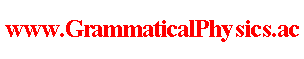since 2006
Help　Sitemap
< Forum >
< Problems >
< Quantum History Theory >

Quantum history theory is one of the nearest trial to produce some concrete physical theory as speedily as possible.

 New Quantum Grammar Measure of Functional Integral Discretized Quantum Mechanical Model Discretized Quantum Mechanical Solution Translation Invariance Probability Formula in New Grammar Line Integral in Function Space Twist Remove Normalization Examine Probability Formula Application of the Stacked Daruma Game Formula Exact Solution of Uda Equation (2a) Exact Solution of Uda Equation (2b) Exact Solution of Uda Equation (3a) Energy Representation of Uda Equation Number Representation of Uda Equation Uda Equation of Dirac Field Uda Equation of QED Uda Equation of Dirac Particle